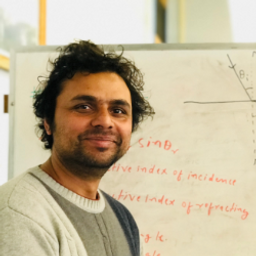Ask your homework questions to teachers and professors, meet other students, and be entered to win \$600 or an Xbox Series X 🎉Join our Discord!University of Wisconsin - Milwaukee

# A jeweler’s lens of focal length 5.0 cm is used as a magnifier. With the lens held near the eye, determine (a) the angular magnification when the object is at the focal point of the lens and (b) the angular magnification when the image formed by the lens is at the near point of the eye (25 cm). (c) What is the object distance giving the maximum magnification?

## a. 5.0b. 6.0c. 4.17 \mathrm{cm}

#### Topics

Electromagnetic Waves

Wave Optics

### Discussion

You must be signed in to discuss.
##### Top Physics 103 Educators##### Marshall S.

University of Washington##### Jared E.

University of Winnipeg

### Video Transcript

So this problem we have the focal length of the magnifier is five centimeter. So in part A When the object is placed at the focal length or a focal point, the eye is relaxed and the image will form at the infinity and the angler magnification. In this case, if you follow the textbook in the angler, magnification and excuse will be 25 centimeter over f. Okay, so this is very straightforward. 25/5, which is going to be five now for part B. When the image is formed at the normal near point, which is cuticles negative 25 centimeter. This is when the angular magnification is actually going to be a maximum. And in this case, the angler magnification is going to be M. Max because one plus 25 centimeter over f and this is going to be one plus. You can just follow from part a 25 centimeter over F five and this is gonna be a six. So the maximum magnification is six. So for Parsi now for maximum magnification using cuticles negative 25 centimeter. And we know the focal length is five. Senator, we can just write down p, which is the object distance as que times half over Q minus F and this is going to be 25 negative 25 times focal length, which is five over negative 25 minus five. This will give me a 4.17 centimeter, which is the object distance in this case.University of Wisconsin - Milwaukee

#### Topics

Electromagnetic Waves

Wave Optics

##### Top Physics 103 Educators##### Marshall S.

University of Washington##### Jared E.

University of Winnipeg